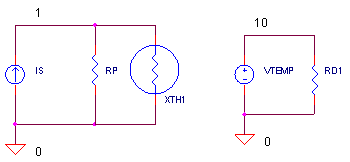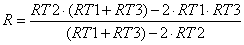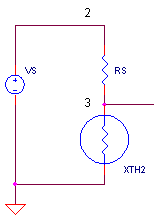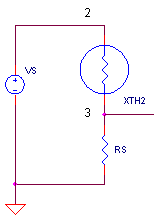eCircuit  Center

### Linearized NTC Thermistor

CIRCUITThermistors offer a distinct advantage over other temperature sensors - high sensitivity! But thermistors come with a price. The resistance versus temperature curve is highly non-linear. However, a simple technique can straighten out the bow in the curve, at least over a reasonable temperature range. Although this technique will reduce the sensitivity of the sensor, the benefit of a linear response is typically worth the cost.

For a look inside the device's SPICE model, see NTC Thermistor Model. ( NTC =  Negative Temperature Coefficient - the resistance decreases as temperature increases. )

PARALLEL RESISTOR

Suppose you've been asked to design a linearized thermistor circuit, with a temperature range centered around +25 deg C. One technique is easy - place a resistor in parallel with the NTC thermistor. But first, to get a feel for the R vs. T curve, check out the table below.

 T (deg C) R (Ohms) -15 63338 +25 10000 +65 2444

This represents a typical thermistor defined by a resistance of R = 10k Ω at 25 ° C having a material constant Beta = 3450.

HANDS-ON DESIGN    Run a simulation of THERM_CKT1.CIR. Current source IS drives the parallel combination of thermistor XTH1 and resistor RP. Initially, we set RP = 1000 kΩ so it has little effect on the thermistor. Plot V(1) to see the voltage across XTH1. VTEMP generates a voltage that really represents the temperature sweeping from -15 to +65 ° C.  ( To see the temperature, plot V(10) in another window.) Although a meager 0.1 mA is supplied by IS, a hefty voltage is produced by the thermistor. But, as you can see - the curve isn't pretty.

Before you place the magic RP into the circuit, you need to ask yourself what value? Find out by trying a few values like RP = 100 k, 10 k and 1 kΩ. Which value pulls the curve the straightest? Looks like a value close to thermistor's value at +25 °C works pretty well. One method calculates an optimal RP as followswhere RT1, RT2 and RT2 are the thermistor's resistance at the low, middle and high temperature points of your measurement range, T1, T2 and T3.

SERIES RESISTOR

But what if there's no budget for a current source on your circuit. And to boot, voltage sources are already available on the circuit board. How can we make use of this voltage source? Recall that a wise man named Thevenin found that a current source in parallel with a resistor is a close cousin of a voltage source in series with a resistor. To make use of this knowledge, replace IS and RP with VS and RS.HANDS-ON DESIGN    Run a simulation of THERM_CKT1.CIR. Voltage source VS and series resistor RS form a voltage divider with thermistor XTH2. Initially, RP = 1000k. Plot V(3) to see the voltage divider output. Run a few simulations to find out which value, RS = 100k, 10k or 1k Ω, pulls the curve the straightest.  You can use the same formula above to calculate an optimal value of RS.

POSITIVE TEMPERATURE COEFFICIENT

Wouldn't it be nice if you could turn the negative temperature coefficient into a positive one? The voltage source and series resistor makes it easy. Just swap the locations of RS and XTH2!How does this change the slope of the output signal? Remember, as the temperature goes up, the thermistor's resistance gets smaller. And because it's now in the upper leg of the divider, the output voltage increases.

HANDS-ON DESIGN    Interchange the positions of RS and XTH2. The netlist should look like

XTH2 2 3 10 0 NTC_10K_1
RS 3 0 10K

Run a simulation of THERM_CKT1.CIR and  plot V(3). Has the output curve been transformed into a positive temperature coefficient?

SPICE FILE

Download the file or copy this netlist into a text file with the *.cir extention.

```THERM_CKT1.CIR - LINEARIZED THERMISTOR
*
* TEMPERATURE
VTEMP	10	0	PWL(0MS -15DEG	100MS 65DEG)
RD1		10	0	1MEG
*
* CURRENT SOURCE AND PARALLEL RESISTOR
IS	0	1	DC	0.1MA
RP	1	0	1000K
XTH1	1 0  10 0   NTC_10K_1

*
* VOLTAGE SOURCE AND SERIES RESISTOR
VS	2	0	DC	10
RS	2	3	1000K
XTH2	3 0  10 0   NTC_10K_1
*
*
* THERMISTOR SUBCIRCUIT ************************
*
* thermistor terminals:	1,2
* temperature input+,-:	4,5
* (temperature in deg C)
*
.SUBCKT NTC_10K_1   1  2  4  5
ETHERM	1 3 VALUE={i(VSENSE)*10K*EXP(3450/(V(4,5)+273.15)-3450/(25+273.15)) }
VSENSE	3	2	DC	0
.ENDS
*
*
* ANALYSIS
.TRAN 	0.1MS  100MS
* VIEW RESULTS
.PRINT	TRAN 	V(1)
.PROBE
.END```

top ↑